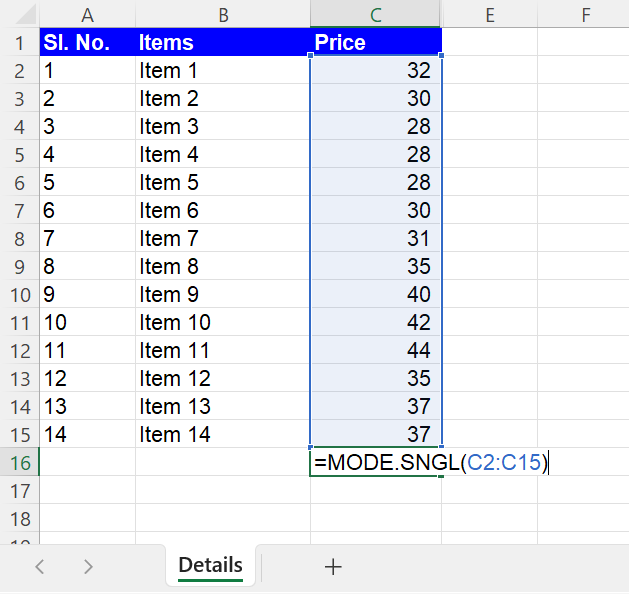Excel Formulas

# MODE Function in Excel (Easy Guide to Understand MODE.SNGL and MODE.MULT)

This article will guide you on how to compute mode in Excel by utilizing MODE.SNGL and MODE.MULT formulas.

## The MODE formula in Excel

The MODE function in Excel is used to determine the mode of chosen values, essentially providing the most frequently occurring value in the selected data.

## When can the MODE function in Excel be used?

The MODE formula can be useful in data analysis when trying to identify the most common value or when working with categorical data. Here are two examples of when the MODE formula can be used in Excel:

1. In a sales report, you can use the MODE function to find the most commonly sold product. For example:  if you have a list of sales data that includes the names of products sold, you can assign a unique numeric value to each product and use the MODE function to find the product that was sold most frequently.
2. In a grade book, you can use the MODE formula to find the most commonly earned grade among the students.

Owing to the limitations of the MODE formula, it is recommended to use the MODE.SNGL and MODE.MULT functions in Excel.

## What are MODE.SNGL and MODE.MULT formulas in Excel?

The MODE.SNGL and MODE.MULT functions are both used to find the mode in a range of values in Excel, but they differ in their output and the types of data they can handle.

The MODE.SNGL function returns only a single mode from the range of data, which is the most commonly occurring value. If there are multiple values with the same frequency of occurrence, the function will return the value that occurs first in the sequence of the selected data points. For example: “=MODE.SNGL(1,2,1,2)” will calculate the mode as 1 and “=MODE.SNGL(2,1,1,2)” will calculate the mode as 2. This function is useful when there is a single mode in the data set, or when only one mode needs to be considered.

The MODE.MULT function, on the other hand, returns an array of all the modes in the range of data. This function is useful when there are multiple modes in the data set, or when you need to consider all the modes.

If there is only one mode in the range of data, the MODE.MULT function will return the same result as the MODE.SNGL function. Additionally, the MODE.SNGL and MODE.MULT can handle non-numeric data, such as text, but error values such as #N/A will return an error.

In summary, the main difference between the MODE.SNGL and MODE.MULT formulas in Excel is:

The MODE.SNGL function returns a single mode while the MODE.MULT function returns an array of modes. In the case of MODE.MULT, the array of modes will be displayed vertically. Hence, you need to ensure that there are enough blank cells below the cell where the MODE.MULT formula is inserted.

## How to insert the MODE.SNGL and MODE.MULT functions in Excel

Step 1: Enter “=MODE.SNGL(” or “=MODE.MULT(” into the desired cell to calculate the mode

Step 2: Choose the values one by one, separating them with “,” (commas), or select an array of data as shown in the image belowImage illustrating the use of the MODE.SNGL formula to select an array of data in Excel

Step 3: Press the “ENTER” key

## Analyze your live financial data in a snap in Google Sheets

Are you learning this formula to visualize financial data, build a financial model, or conduct financial analysis? In that case, LiveFlow may help you automate manual workflows, update numbers in real-time, and save time. You can access various financial templates on our website, from the simple Income Statement to Multi-Currency Consolidated Financial Statement. Are you interested in this product but are an Excel user? That’s not a problem at all. You can connect Google Sheets to Excel quickly.